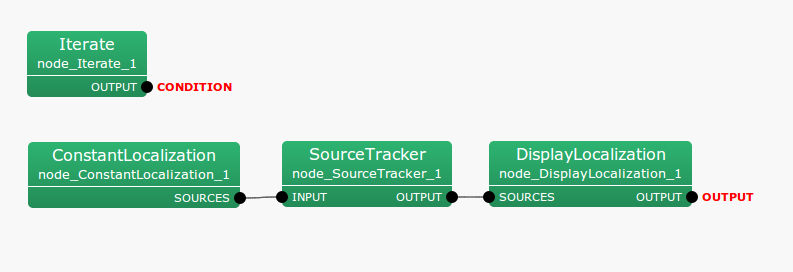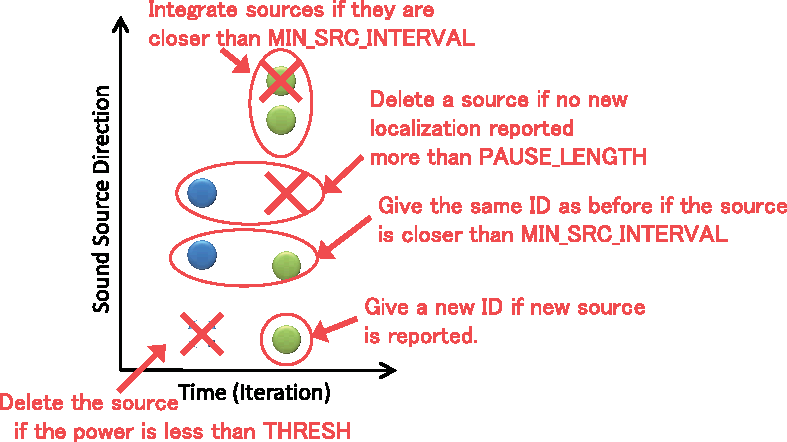## 6.2.19 SourceTracker

### 6.2.19.1 Outline of the node

For source localization results input in time series without IDs, this node gives the same ID to the source localization result obtained from an adjacent direction and a different ID to source localization results obtained from different directions. After running this node, the user can judge if the sound sources are same by IDs.

### 6.2.19.2 Necessary file

No files are required.

### 6.2.19.3 Usage

When to use

Source localization results vary even though sound sources are fixed (e.g. standing person or fixed speaker). Usually, they are not obtained from the same direction continuously. Therefore, in order to unify source location results so that they can be treated as coming from the same sound source, it is necessary to track the source location results. SourceTracker  uses an algorithm that gives the same ID to source localization results when sound sources are sufficiently close. As a criterion for judging if the sound source is sufficiently close to another, the user may set an angle as a threshold. IDs are given to sound sources with this node, which enables to perform processing for each ID.

Typical connection

Usually, the outputs of source localization nodes such as ConstantLocalization  or LocalizeMUSIC  are connected to the input terminal of this node. Then an appropriate ID is added to a localization result so users can connect it to the sound source separation module GHDSS  or the presentation node for source location results (DisplayLocalization ), which are based on source localization. Figure 6.47 shows a connection example. Here, a fixed source location result is displayed through SourceTracker . In this case, if the localization result that ConstantLocalization  outputs is close to another, they are output together in one sound source. When giving the following property to ConstantLocalization  in the figure, the angle between the two sound sources is less than 20[deg], which is the default value of MIN_SRC_INTERVAL and therefore only one sound source is presented.

ANGLES:
ELEVATIONS:

See ConstantLocalization  for the meaning of the set pointsFigure 6.47: Connection example of SourceTracker

### 6.2.19.4 Input-output and property of the node

Input

INPUT

: Vector<ObjectRef>  type. Source localization result with no ID given.

Output

OUTPUT

: Vector<ObjectRef>  type. Source localization result for which the same ID is given to sound sources positioned near to another

Parameter

Table 6.34: Parameter list of SourceTracker
 Parameter name Type Default value Unit Description THRESH To be ignored if the MUSIC power of the sound source is smaller than THRESH. PAUSE_LENGTH 800 [frame*10] Length when assuming that the localized sound continues. COMPARE_MODE DEG DEG or TFINDEX Method for comparing inter-source distance. If the value is DEG, they are calculated using triangular functions. If the value is TFINDEX, they are calculated using index comparison. MIN_SRC_INTERVAL 20 [deg] Threshold value of angular difference for judging that the sound source is same as another. (Valid if COMPARE_MODE = DEG) MIN_TFINDEX_INTERVAL 3 Threshold value of index difference for judging the sound source is same as another. (Valid if COMPARE_MODE = TFINDEX) MIN_ID 0 DEBUG false
THRESH

: float  type. This parameter judges by the MUSIC power whether the source localization result is noise to be ignored. The result is considered noise if the MUSIC power is smaller than THRESH, and the localization result is not sent to the output. When THRESH is too small, noise is sent to output, and when it is too large, it becomes difficult to localize the target sound, and therefore it is necessary to find the value that meets this trade-off.

PAUSE_LENGTH

: float  type. This parameter determines how long the sound source once output as a localization result. For a direction that is localized once, even though there are no valid source localization results after the first localization, localization results for that direction continue being output during a period of PAUSE_LENGTH / 10 [frame]. Since the default value is 800, for a direction that is localized once, localization results continue being output for 80 [frame] after the first localization.

COMPARE_MODE

:  type. If the value is DEG, comparison is done using triangular functions. If the value is TFINDEX, comparison is done by index comparison. Since triangular function is heavier, TFINDEX reduces the computation time. TFINDEX is useful only if the index difference and angular difference are equivalent, i.e., transfer functions are recorded in order.

MIN_SRC_INTERVAL

: float  type. If the source location result is smaller than MIN_SRC_INTERVAL, the two sound sources are judged as an identical sound source, and the influence of fluctuating motion of source localization is reduced by deleting either source localization result. This parameter is valid if COMPARE_MODE = DEG.

MIN_TFINDEX_INTERVAL

: int  type. Almost the same as MIN_SRC_INTERVAL. The difference is that the compared values are not angles but indexes. This parameter is valid if COMPARE_MODE = TFINDEX.

MIN_ID

:

DEBUG

: bool  type. If this value is true, the localization results are given to stderr.

### 6.2.19.5 Details of the node

Definitions of symbols: First, symbols used in this section are defined.

1. ID: ID of sound source

2. Power $p$: Power of the direction localized.

3. Coordinate $x,y,z$: Cartesian coordinate on a unit ball corresponding to the source localization direction.

4. Duration $r$: The index that assumes how long the localized sound source lasts.

The MUSIC power of the sound source localized is $p$, and the Cartesian coordinates on a unit ball corresponding to the sound source direction are $x$, $y$, $z$. Assuming $N$ is the number of sound sources that a node presently maintains and $M$ is that of the sound sources newly input, they are distinguished by the subscripts $^{last}$ and $^{cur}$. For example, MUSIC power of the $i$ th newly input sound source is indicated as $p^{cur}_ i$. The angle between sound sources, which is an index that judges closeness of the sound sources, is assumed $\theta$.

Criterion of closeness of sound source directions:

Assuming two sound source directions as coordinates of ${\boldsymbol q}_1 = (x_1, y_1, z_1)$ and ${\boldsymbol q}_2 = (x_2,y_2,z_2)$, the angle $\theta$ is expressed as follows.

 \begin{equation} {\boldsymbol q}_1 \cdot {\boldsymbol q}_2 = |{\boldsymbol q}_1| |{\boldsymbol q}_2| \cos \theta \end{equation} (17)

Then, $\theta$ is obtained by applying an inverse trigonometric function.

 \begin{equation} \theta = \cos ^{-1} \left( \frac{ {\boldsymbol q}_1 \cdot {\boldsymbol q}_2 }{ |{\boldsymbol q}_1| |{\boldsymbol q}_2| } \right) = \cos ^{-1} \left( \frac{ x_1\cdot x_2 + y_1 \cdot y_2 + z_1 \cdot z_2 }{ \sqrt { x_1^2 + y_1^2 + z_1^2 } \sqrt { x_2^2 + y_2^2 + z_2^2 } } \right) \end{equation} (18)

In order to simplify the indication, the angle between the $i$th sound source and the $j$th sound source is expressed as $\theta _{ij}$ below.

Sound source tracking method:

The processing that SourceTracker  performs in sound source tracking is shown in Figure 6.48. In the figure, the horizontal axis indicates (=repeat count) and the vertical axis indicates sound source directions. Moreover, the blue circle indicates the source position ($^{last}$) that the node already has and the green circle indicates the source location ($^{cur}$) newly input. First, for all the sound sources, if the MUSIC powers $p^{cur}_ i and p^{last}_ j$ are smaller than THRESH, they are deleted. Next, comparing the source positions newly input with the localization information that the node already has, if they are sufficiently close (=$\theta _{ij}$ is below MIN_SRC_INTERVAL[deg]), they are integrated. The same ID is given to the integrated sound sources and the duration $r^{last}$ is reset at PAUSE_LENGTH. If source positions newly input are sufficiently close, they are integrated to one sound source. Integration is achieved by leaving one sound source and deleting the other sound positions. The sound sources with $\theta _{ij}$ larger than MIN_SRC_INTERVAL [deg] are judged as different sound sources. For the source positions that the node already has but are not newly input, $r^{last}$ is reduced by ten. The sound sources with $r^{last}$ less than zero is judged to have disappeared and is deleted. If the source position newly input is different from any of those that the node already has, a new ID is given to the sound source and $r^{cur}$ is initialized at PAUSE_LENGTH.Figure 6.48: Sound source tracking method of SourceTracker